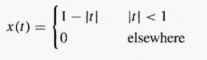# Let X T Be A Triangular Pulse Defined By A By Taking The Derivative Of X T Use The D 2298035

Let x(t) be a triangular pulse defined by(a) By taking the derivative of x(t), use the derivative property to find the Fourier transform of x(t).

Express the derivative as a sum of two pulses. one with an amplitude of one, the other with an amplitude of minus one. From the tables of Fourier transform pairs and properties, write down the Fourier transform of the derivative of x(t). Then, use the derivative property in the frequency-domain to find X(jω).

(b) Find the Fourier transform of x(t) by differentiating x(t) twice and using the second-derivative property. Compare this result to the result of part (a).4.6/5

Price (USD)
\$